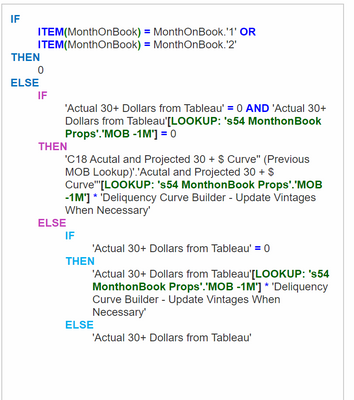# Recreating Excel Formula in AnaplpanHi,

Trying to replicate this same Excel Formula in Anaplan, but leads into a Circular Reference. Please advise on how would do so. The Excel Formula and the Formula we are attempting to us in Anaplan are below.

Excel Formula: =IF(AND(AJ31=0,AK31=0),AJ103*AK\$71,IF(ISBLANK(AK31),AJ31*AK\$71,AK31))

Anaplan Formula:Thank you

Tagged: